2.4.2 Distribution

The purpose of a distribution step is to allow the search to proceed by making a non-deterministic choice.

Naive Strategy

The naive distribution strategy for integer variables picks a non-determined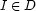and non-deterministically infers either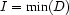or. For finding solutions of 2*A=B with A and B integers between 0 and 9 inclusive, the strategy produces the search tree below: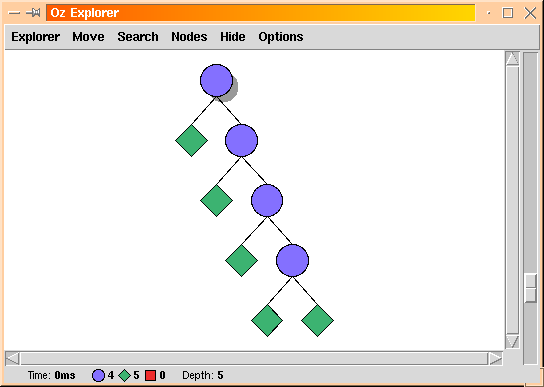Domain Splitting

Another strategy for integer variables is known as domain splitting. It picks a non-determined integer variable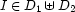, whereand non-deterministically infers either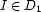or. For the same problem 2*A=B this strategy produces the search tree below:Alternating Steps of Propagation and Distribution

The picture below shows a propagation step followed by a distribution step that creates a branching point in the search tree. The distribution strategy used is domain splitting on variable: each branch investigates one half of's domain: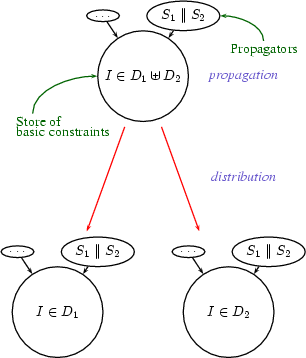Denys Duchier
Version 1.2.0 (20010221)